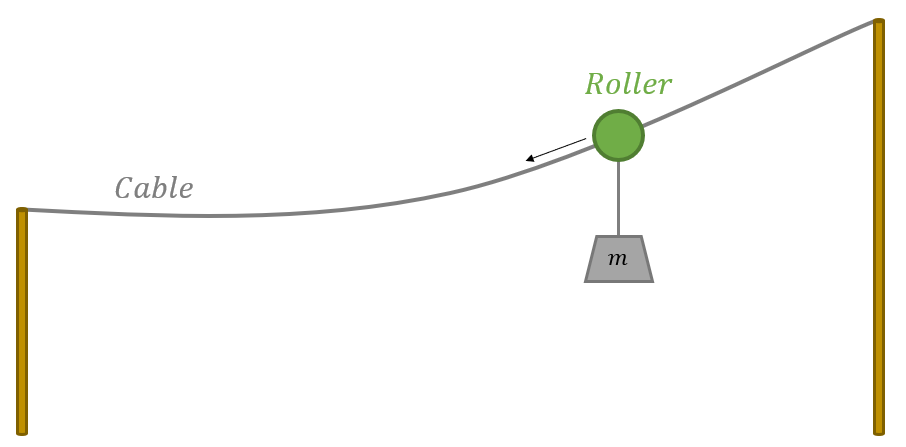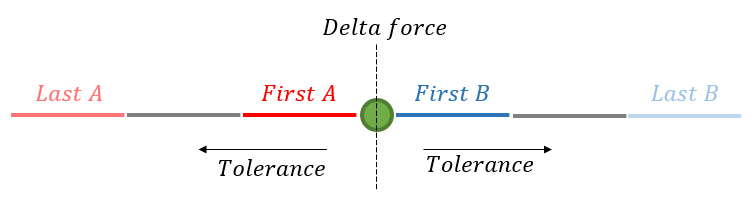# Roller

### Prerequisites

The tutorial presents a simple case study with the purpose of demonstrating functionality in AquaSim.

It is assumed that the user is familiar with the basic principles of modelling and specifying material parameters in AquaEdit, as well as conducting analyses. If you are looking for an introduction to AquaSim we advise you to start with the Basic program tutorials.

### Learning objectives

Upon completion of this case study, you will be able to:

• Understand the fundamentals of a roller in AquaSim
• Specify roller parameters
• Run static analysis with roller

### Introduction

In this case study you will establish a system with a roller. Attached to the roller is a rope with mass. Due to gravitational forces, the roller will slide along the cable. Further, you are going to assess the forces in the cable when adjusting the key-parameters for a roller.### Principles of roller

In this section, the principles of roller in AquaSim are explained. Rollers are typically applied for ropes to slide along other ropes. A principle sketch of how roller is defined is given in the figure below.In AquaSim, rollers are applied to nodes as a node decorator. To define the range where the roller can slide a first- and last-element is defined on each side of the roller. The first-elements A and B are automatically selected by AquaSim. The last-elements are where the roller will stop and can be edited by the user. When an analysis is run, AquaSim will compare the axial forces between A and B and adjust the length of the elements so that the forces are within the defined criteria. The analysis timestep will not converge until one or more of these criteria are satisfied.

The Delta force may be interpreted as a frictional resistance in the roller. When Delta force is assigned positive values, element A should have higher axial force than element B. When Delta force is assigned negative values, element B should have higher axial force than element A.

Having defined a value for both Delta force and Tolerance, the timestep will not converge until the axial forces are within the Tolerance of the defined Delta force. If Delta force is 0, then the timestep will converge when the axial force between element A and B are lower than the defined Tolerance.